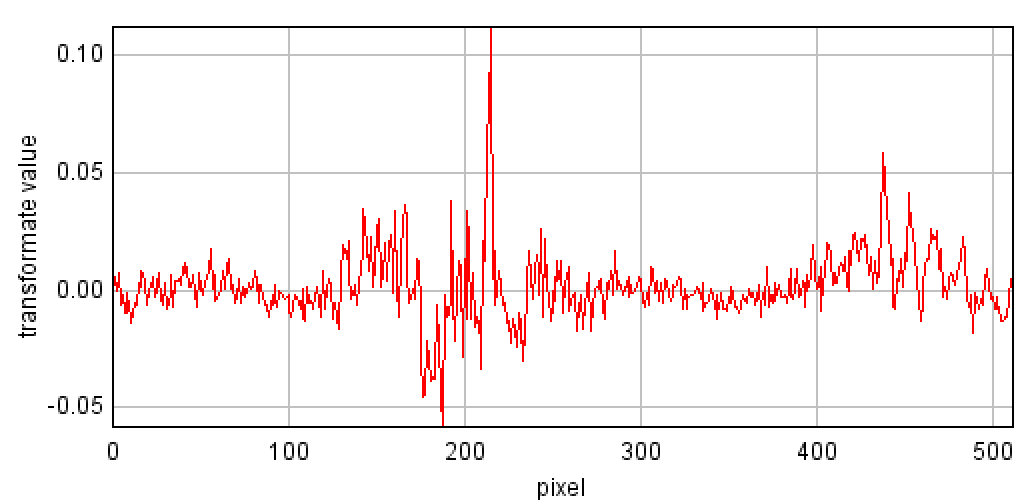# Ambiguous results of phase correlation in pattern recognition

We are using phase correlation to find pattern on image and what we get is 15% of results is spurious. Ours pattern has 32x32 pixels and image for research has 32x512 pixels. So we put Pattern to 32x512 pixels image to the left side and the rest of image is filled by zero pixels. Next we apply FFT to pattern and the image without applying a window function. Then we make Hadamard product, normalized it and do inverse FFT of resulting matrix. Sometimes we get false peak almost on the middle of plot where edge effects and circularity of correlation should be neglected.

The question:

What else could be the cause of false peak ?

Images:

what was searched and what we got:real research image 32x512 (before greyscale)real pattern in (in greyscale)correlation resultBest Regards

Dawid D

• I'm working in frequency domain and I can't find how is defined phase correlation in spatial domain so i don't fully understand the math behind the code. @lennon310 can you explicitly write the equations ? Thank you very much for your help. – user7583 Jan 17 '14 at 8:10
• @deadline, my code works on spatial domain. It's a normalized cross correlation, you can check this paper for reference: scribblethink.org/Work/nvisionInterface/nip.pdf Thanks – lennon310 Jan 17 '14 at 15:51

I suspect your problem occurs due to some scaling issues. Basically you need to normalize your research image to the pattern template by subtracting the mean value of the template. And it is better calculate the ratio of correlation to the standard deviation of both images.

I don't know which programming language you are using. I wrote a Matlab code for you to better get what I meant above (note that I did not use zero padding since I work on the spatial domain, I don't think it is the issue that caused your spurious correlation too):

file1='https://lh3.googleusercontent.com/-GQSrln7qnO8/UtCT8elB_FI/AAAAAAAAEaw/bh7aKyyMRIo/s512/subimage.png'; % Research image

It=double(I2);  % template
Ii=double(I1);  % image

Ii_mean = conv2(Ii,ones(size(It))./numel(It),'same');
It_mean = mean(It(:));
corr_1 = conv2(Ii,rot90(It-It_mean,2),'same')./numel(It);
corr_2 = Ii_mean.*sum(It(:)-It_mean);
conv_std = sqrt(conv2(Ii.^2,ones(size(It))./numel(It),'same')-Ii_mean.^2);
It_std = std(It(:));
S = (corr_1-corr_2)./(conv_std.*It_std);

imagesc(abs(S))


What you get:You can observe the correlation peak at 17th row, 450th column, which is the position of your pattern in original image.

You can also use Matlab built-in function normxcorr2(It,Ii);, just note that this function results in the correlation map with the sum of the lengths of two images, while my code provides you the correlation map the same size of your research image.

• I think he does need to do zero padding if he wants to do FFT based convolution. You got away with not having it by using conv2 instead. – Aaron Jan 13 '14 at 17:20
• @Aaron thanks Aaron. Yes I found that zero padding may not a big issue, it is probably mainly due to the scaling problem. I deleted that part in my answer. Thanks – lennon310 Jan 13 '14 at 17:43
• @lennon310 Thank you for your answer. We are using ImageJ so whole ours code is in Java. We are implementing division by standard driviation in the sumtable schema because there is a lot of calculations. I only doesn't understand corr_2 line there will by zero always becouse sum of element with subtrac mean value will always zero. Can an you explain please, why are you multiplying mean value of image by sum of template value with subtract mean value? – Dawid D Jan 17 '14 at 15:15
• @DawidD thank you very much for pointing out! You are right we don't need corr_2 since it is always zero. My code is just a normalized cross correlation (scribblethink.org/Work/nvisionInterface/nip.pdf), and there is no corr_2 either. Thank you:) – lennon310 Jan 17 '14 at 15:37